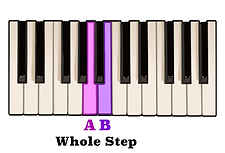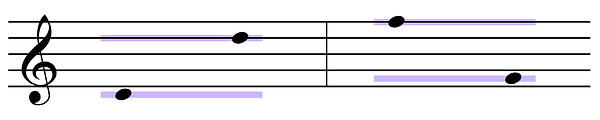top of page

## Interval Identification | Perfect Eighths And Octaves (8 of 10)

An interval of a Perfect eighth, also known as an octave, is an interval in which two notes of equal letter name are sounded at double, or half, the vibrational frequency of each other.

For example, if a note vibrates at a frequency of approximately 440 hertz, or 440 cycles per second, then an octave above that note will vibrate at a frequency of approximately 880 cycles per second while an octave below that note vibrates at a frequency of about 220 cycles per second.

The amount of steps in between any two pitches which produces an interval of a Perfect eighth is any combination of steps equalling twelve consecutive half steps. Twelve half steps can also be looked at as being five whole steps and two half steps.

Let's use this pattern of steps to find an interval of a perfect eighth above the note C.One whole step above the note C is the note D.A whole step above the note F is the note G.A half step above D is the note E.Another whole step above G is the note A.The notes E and F are separated by a half step.One more whole step above the note A is the note B.And a half step above the note B is C.We can see that an interval of a Perfect eighth above C is the next C.

Because of the amount of steps separating each note, identifying an interval of a Perfect eighth on the staff can be a bit tricky. However, you will notice that if the bottom note of the octave is on a line then the top note will always be found on a space with three lines and three spaces between them.And if the bottom note is on a space then the top note will be found on a line with three spaces and three lines between them.However, the easiest way to identify this interval would be to simply read the letter name of each note. If the letter names are the same then you will know that the interval is a Perfect eighth, or octave.In previous videos in which we discussed an interval of a Perfect fourth and an interval of a Perfect fifth we learned that any interval which is considered Perfect is always the same note position on both a Major and minor scale.

This applies to an interval of a Perfect eighth as well. An interval of a Perfect eighth is simply the root note found after the seventh scale degree of that note's Major or minor scale.bottom of page